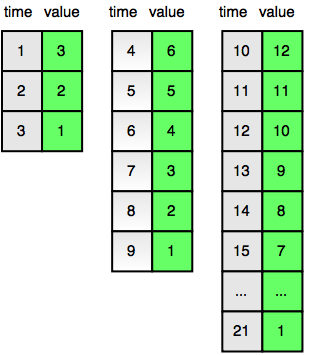# Strange Counter Hackerrank problem solution

##### Strange Counter Hackerrank

Bob has a strange counter. At the first second, t=1, it displays the number 3. At each subsequent second, the number displayed by the counter decrements by 1.

The counter counts down in cycles. In the second after the counter counts down to 1, the number becomes 2 X the initial number for that countdown cycle and then continues counting down from the new initial number in a new cycle. The diagram below shows the counter values for each time t in the first three cycles:Given a time, t, find and print the value displayed by the counter at time t.

Input Format

A single integer denoting the value of t.

Constraints
1<=t<=1012
1<=t<=106
for 60% of the maximum score.
Output Format

Print the value displayed by the strange counter at the given time t.

Sample Input

4
Sample Output

6
Explanation

Time t=4 marks the beginning of the second cycle in which the counter displays a number that is double the initial number displayed at the beginning of the previous cycle (i.e., 2 X 3=6). This is also shown in the diagram in the Problem Statement above.

##### Solution
```#include <iostream>

using namespace std;

long long n;

int main(){

cin >> n;
long long P = 3;
while (n > P)
{
n -= P;
P *= 2;
}
cout << P - n + 1 << endl;

return 0;
}
```
``````import java.io.*;
import java.util.*;
import java.text.*;
import java.math.*;
import java.util.regex.*;
public class StrangeCounter {
public static void main(String[] args) {
Scanner in = new Scanner(System.in);
long t = in.nextLong();
long d = 3;
long ans = 0;
while(2>1){
if(t<=d){
ans = d+1-t;
break;
}
else{
t-=d;
d  =  2*d;
}
}
System.out.println(ans);
}
}``````
``````time = input()
t = 1
value = 3
delta = 3
while (t + delta <= time):
t += delta
delta *= 2
value *= 2
print value - time + t``````

### hasectic

A web developer(Front end and Back end), and DBA at csdamu.com. Currently working as Salesforce Developer @ Tech Matrix IT Consulting Private Limited. Check me @about.me/s.saifi